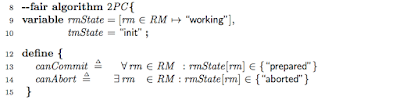## Wednesday, January 18, 2017

### Deep Learning With Dynamic Computation Graphs (ICLR 2017)

This is a paper by Google that is under submission to ICLR 2017. Here is the OpenReview link for the paper. The paper pdf as well as paper reviews are openly available there. What a concept!

This paper was of interest to me because I wanted to learn about dynamic computation graphs. Unfortunately almost all machine learning/deep learning (ML/DL) frameworks operate on static computation graphs and can't handle dynamic computation graphs. (Dynet and Chainer are exceptions).

Using dynamic computation graphs allows dealing with recurrent neural networks (RNNs) better, among other use cases. (Here is a great article about RNNs and LSTMs. Another good writeup on RNNs is here.) TensorFlow already supports RNNs, but by adding padding to ensure that all input data are of the same size, i.e., the maximum size in the dataset/domain. Even then this support is good only for linear RNNs not good for treeRNNs which is suitable for more advanced natural language processing.

This was a very tough paper to read. It was definitely above my level as a beginner. The paper assumed a lot of background from the reader. It assumed familiarity with TensorFlow execution and operators, and also some understanding of programming language background and familiarity with RNNs. The dynamic batching idea introduced in the paper is a complex idea but it is explained briefly (and maybe a bit poorly?) in one page. Even when I gave the paper all my attention, and tried to form several hypothesis of dynamic batching idea, I was unable to make progress. At the end, I got help from a friend who is an expert at deep learning.

I skipped reading the second part of the paper which introduced a combinator library for NNs. The library is relevant because it was instrumental in implementing the dynamic batching idea introduced in the first part of the paper. This second part looked interesting but the functional programming language concepts discussed was hard for me to follow.

## The dynamic batching idea

This paper introduces dynamic batching idea to emulate dynamic computation graphs (DCGs) of arbitrary shapes and sizes over TensorFlow which only supports static computation graphs.

Batching is important because GPUs crave for batching, especially when dealing with text data where each item is of small size. (While images are already large enough to fill/busy the GPU, but that is not so for text data.)

However, the challenge for batching when using DCGs is that the graph of operations is not static, and can be different for every input. The dynamic batching algorithm fixes batching for DCGs. Given a set of computation graphs as input, each of which has a different size and topology, dynamic batching algorithm will rewrite the graphs by batching together all instances of the same operation that occur at the same depth in the graph. (Google is really into graph rewriting.)

The dynamic batching algorithm takes as input a batch of multiple input graphs and treats them as a single disconnected graph. Source nodes are constant tensors, and non-source nodes are operations. Scheduling is performed using a greedy algorithm: (I omit some of the more detailed steps in the paper.)
• Assign a depth, d, to each node in the graph. Nodes with no dependencies (constants) are assigned depth zero. Nodes with only dependencies of depth zero, are assigned depth one, and so on.
• Batch together all nodes invoking the same operation at the same depth into a single node.
• Concatenate all outputs which have the same depth and tensor type. The order of concatenation corresponds to the order in which the dynamic batching operations were enumerated.
Each dynamic operation is instantiated once in the static dataflow graph. The inputs to each operation are tf.gather ops, and the outputs are fed into tf.concat ops. These TensorFlow ops are then placed within a tf.while_loop. Each iteration of the loop will evaluate all of the operations at a particular depth. The loop maintains state variables for each tensor type t, and feeds the output of concat for tensor type t and iteration d into the input of the gathers at tensor type t and iteration d+1.

## Experimental results

The test results emphasize the importance of batching, especially on GPUs where it can enable speed ups up to 120x. The speedup ratio denotes the ratio between the per-tree time for dynamic batching on random shapes ("full dynamic"), versus manual batching with a batch size of 1.

Dynamic batching instantiates each operation only once, and invokes it once for each depth, so the number of kernel invocations is log(n), rather than n, where n is tree size. Dynamic batching thus achieves substantial speedups even at batch size 1, because it batches operations at the same depth within a single tree.

## Limitations

Dynamic batching works on a single machine, it is not distributed. Dynamic batching requires an all to all broadcasts, so it doesn't scale to distributed machines.

This Google paper doesn't cite or talk about Dynet and Chainer, but Dynet and Chainer are single machine ML/DL frameworks that support dynamic computation graphs. On one hand, Dynet & Chainer are most likely not good at batching, and the dynamic batching method here has contribution. On the other hand, since Dynet & Chainer support dynamic computation graphs natively (rather than by way of emulating it on static computation graphs like dynamic batching does), they are most likely more expressive than the dynamic batching can achieve. In fact, another limitation of the dynamic batching approach is that it requires all operations that might be used to be specified in advance. Each input/output may have a different type but all types must be fixed and fully specified in advance.

## Tuesday, January 17, 2017

### My first impressions after a week of using TensorFlow

Last week I went through the TensorFlow (TF) Tutorials here. I found that I hadn't understood some important points about TensorFlow execution, when I read the TensorFlow paper. I am noting them here fresh to capture my experience as a beginner. (As one gathers more experience with a platform, the baffling introductory concepts starts to occur obvious and trivial.)

The biggest realization I had was to see a dichotomy in TensorFlow among two phases. The first phase defines a computation graph (e.g., a neural network to be trained and the operations for doing so). The second phase executes the computation/dataflow graph defined in Phase1 on a set of available devices. This deferred execution model enables optimizations in the execution phase by using global information about the computation graph: graph rewriting can be done to remove redundancies, better scheduling decisions can be made, etc. Another big benefit is in enabling flexibility and ability to explore/experiment in the execution phase through the use of partial executions of subgraphs of the defined computation graph.

In the rest of this post, I first talk about Phase1: Graph construction, Phase2: Graph execution, and then I give a very brief overview of TensorFlow distributed execution, and conclude with a discussion on visualizing and debugging in TensorFlow.

## Phase1: Graph construction

This first phase where you design the computation graph is where most of your efforts are spent. Essentially the computation graph consists of the neural network (NN) to be trained and operations to train it. Here you lay out the computation/dataflow graph brick by brick using TensorFlow operations and tensors. But what you are designing is just a blueprint, nothing gets built yet.

Since you are designing the computation graph, you use placeholders for input and output. Placeholders denote what type of input is expected. For example, x may correspond to your training data, and y_ may be your training labels, and you may define them as follows using the placeholders.
x = tf.placeholder(tf.float32, [None, 784])
y_ = tf.placeholder(tf.float32, [None, 10])

This says that x will later get instantiated unspecified number of rows (you use 'None' to tell this to TensorFlow) of 784 float32 vectors. This setup enables us to feed the training data to the NN in batches, and gives you flexibility in the graph execution phase to instantiate multiple workers in parallel with the computational graph/NN and train them in parallel by feeding them different batches of your input data.

## Phase2: Graph execution using sessions

After you get the computation graph designed to perfection, you switch to the second phase where the graph execution is done. Graph/subgraph execution is done using sessions. A session encapsulates the runtime environment in which graphs/subgraphs instantiate and execute.

When you open a session, you first initialize the variables by calling "tf.global_variables_initializer().run()". Surprise! In Phase1 you had assigned variables initial values, but those did not get assigned/initialized until you got to Phase2 and called "tf.global_variables_initializer". For example, let's say you asked b to be initialized as a vector of size 10 with all zeros "b = tf.Variable(tf.zeros())" in Phase1. That didn't take effect until you opened a session, and called "tf.global_variables_initializer". If you had typed in "print( b.eval() )" in the first part after you wrote "b = tf.Variable(tf.zeros())", you get an error: " ValueError: Cannot evaluate tensor using eval(): No default session is registered. Use with sess.as_default() or pass an explicit session to eval(session=sess)  ".

This is because b.eval() maps to session.run(b), and you don't have any session in Phase1. On the other hand, if you try print (b.eval()) in Phase2 after you call "tf.global_variables_initializer", the initialization takes effect and you get the output [ 0. 0. 0. 0. 0. 0. 0. 0. 0. 0.].

Each invocation of the Session API is called a step, and TensorFlow supports multiple concurrent steps on the same graph. In other words, the Session API allows multiple calls to Session.run() in parallel to improve throughput. This is basically performing dataflow programming over the symbolic computation graph built in Phase1.

In Phase2, you can open sessions and close sessions to your heart's content. Tearing down a session and reopening a session has several benefits. This way you instruct TensorFlow runtime to forget about the previous values assigned to the variables in the computation graph, and start again with a new slate (which can be useful for hyperparameter tuning). When you close a session you release that state, and when you open a session you initialize the graph again and start from scratch. You can even have multiple sessions open concurrently in theory, and that may even be useful for avoiding variable naming clashes.

An important concept for Phase2 is partial graph execution. When I read the TensorFlow paper first time, I hadn't understood the importance of partial graph execution, but turns out it is important and useful. The API for executing a graph allows the client to specify the subgraph that should be executed. The client selects zero or more edges to feed input tensors into the dataflow, and one or more edges to fetch output tensors from the dataflow. Then the runtime prunes the graph to contain the necessary set of operations.

Partial graph execution is useful in training parts of the NN at a time. However, it is commonly exercised in a more mundane way in basic training of NNs.  When you are training the NN, every K iterations you may like to test with the validation/test set. You had defined those in Phase1 when you define the computation graph, but these validation/test evaluation subgraphs are only included and executed every K iterations, when you ask sess.run() to evaluate them. This reduces the overhead in execution.  Another example is the tf.summary operators, which I will talk about in visualizing and debugging. The tf.summary operators are defined as peripheral operations to collect logs from computation graph operations. You can think of them as an overlay graph. If you like to execute tf.summary operations, you explicitly mention this in sess.run(). And when you leave that out, tf.summary operations (that overlay graph) is pruned out and don't get executed. Mundane it is but it provides a lot of computation optimization as well as flexibility in execution.

This deferred execution model in TensorFlow is very different than the traditional instant-gratification instant-evaluation execution model. But this serves a purpose. The main idea of Phase2 is that, after you have painstakingly constructed the computation graph in Phase1, this is where you try to get as much mileage out of that computation graph.

## Brief overview of TensorFlow distributed execution

A TensorFlow cluster is a set of tasks (named processes that can communicate over a network) that each contain one or more devices (such as CPUs or GPUs). Typically a subset of those tasks is assigned as parameter-server (PS) tasks, and others as worker tasks.

Tasks are run as (Docker) containers in jobs managed by a cluster scheduling system (Kubernetes). After device placement, a subgraph is created per device. Send/Receive node pairs that communicate across worker processes use remote communication mechanisms such as TCP or RDMA to move data across machine boundaries.

Since TensorFlow computation graph is flexible, it is possible to easily allocate subgraphs to devices and machines. Therefore distributed execution is mostly a matter of computation subgraph placement and scheduling. Of course there are many complicating factors: such as heterogeneity of devices, communication overheads, just in time scheduling (to reduce overhead), etc. Google TensorFlow papers mention they perform graph rewriting and inferring of just-in-time scheduling from the computation graphs.

I haven't started delving into TensorFlow distributed, and haven't experimented with it yet. After I experiment with it, I will provide a longer write up.

## Visualizing and debugging

TF.summary operation provides a way to collect and visualize TensorFlow execution information. TF.summary operators are peripheral operators; they attach to other variables/tensors in the computation graph and they capture their values. Again, remember the two phase dichotomy in TensorFlow. In Phase1, you define and describe these TF.summary for the computational graph, but they don't get executed. They only get executed in Phase2 where you create a session, execute the graph, and explicitly mention to execute tf.summary graph as well.

If you use the TF.summary.FileWriter, you can write the values the tf.Summary  operations collected during a sess.run() into a log file. Then you can direct the Tensorboard tool to the log file to visualize and see the computational graph, as well as the how the values evolved over time.

I didn't get much use from the Tensorboard visualization. Maybe it is because I am a beginner. I don't find the graphs useful even after having a basic understanding of how to read them. Maybe they get useful for very very large computation graphs.

The Google TensorFlow whitepaper says that there is also a performance tracing tool called EEG but that is not included in the opensource release.

By clicking on label "mldl" at the end of the post, you can reach all my posts about machine learning / deep learning (ML/DL).

In particular the series below reviews the introductory concepts in ML/DL.
Learning Machine Learning: A beginner's journey
Linear Regression
Logistic Regression
Multinomial Logistic Regression

## Thursday, January 12, 2017

### Google DistBelief paper: Large Scale Distributed Deep Networks

This paper introduced the DistBelief deep neural network architecture. The paper is from NIPS 2012. If you consider the pace of progress in deep learning, that is old and it shows. DistBelief doesn't support distributed GPU training which most modern deep networks (including TensorFlow) employ. The scalability and performance of DistBelief has been long surpassed.

On the other hand, the paper is a must read if you are interested in distributed deep network platforms. This is the paper that applied the distributed parameter-server idea to Deep Learning. The parameter-server idea is still going strong as it is suitable to serve the convergent iteration nature of machine learning and deep learning tasks. The DistBelief architecture has been used by the Microsoft Adam project, Baidu Deep Image, Apache Hama, and Petuum's Bosen. Google, though, has since switched from the DistBelief parameter-server to TensorFlow's hybrid dataflow architecture, citing the difficulty of customizing/optimizing DistBelief for different machine learning tasks. And of course TensorFlow also brought support for distributed GPU execution for deep learning, which improves performance significantly.

I think another significance of this paper is that it established connections between deep-learning and distributed graph processing systems. After understanding the model-parallelism architecture in DistBelief, it is possible to transfer some distributed graph processing expertise (e.g., locality-optimized graph partitioning) to address performance optimization of deep NN platforms.

## The DistBelief architecture

DistBelief supports both data and model parallelism. I will use the Stochastic Gradient Descent (SGD) application as the example to explain both cases. Let's talk about the simple case, data parallelism first.

### Data parallelism in DistBelief

In the figure there are 3 model replicas. (You can have 10s even 100s of model replicas as the evaluation section of the paper shows in Figures 4 and 5.) Each model replica has a copy of the entire neural network (NN), i.e., the model. The model replicas execute in a data-parallel manner, meaning that each replica works at one shard of the training data, going through its shard in mini-batches to perform SGD. Before processing a mini-batch, each model replica asynchronously fetches from the parameter-server service an update copy of its model parameters $w$. And after processing a mini-batch and computing parameter gradients, $\Delta w$, each model replica asynchronously pushes these gradients to the parameter-server upon which the parameter-server applies these gradients to the current value of the model parameters.

It is OK for the model replicas work concurrently in an asynchronous fashion because the $\Delta$ gradients are commutative and additive with respect to each other. It is even acceptable for the model replicas to slack a bit in fetching an updated copy of the model parameters $w$. It is possible to reduce the communication overhead of SGD by limiting each model replica to request updated parameters only every nfetch steps and send updated gradient values only every npush steps (where nfetch might not be equal to npush). This slacking may even be advantageous in the beginning of the training when the gradients are steep, however, towards converging to an optima when the gradients become subtle, going like this may cause dithering. Fortunately, this is where Adagrad adaptive learning rate procedure helps. Rather than using a single fixed learning rate on the parameter server, Adagrad uses a separate adaptive learning rate $\eta$ for each parameter. In Figure 2 the parameter-server update rule is $w' := w - \eta \Delta w$.  An adaptive learning with large learning rate $\eta$ during convergence, and small learning rate $\eta$ closer to the convergence is most suitable.

Although the parameter-server is drawn as a single logical entity, it is itself implemented in a distributed fashion, akin to how distributed key value stores are implemented. In fact the parameter server may even be partitioned over the model replicas so each model replica becomes the primary server of one partition of the parameter-server.

### Model parallelism in DistBelief

OK now to explain model-parallelism, we need to zoom in each model replica. As shown in the Figure, a model-replica does not need to be a single machine. A five layer deep neural network with local connectivity is shown here, partitioned across four machines called model-workers (blue rectangles). Only those nodes with edges that cross partition boundaries (thick lines) will need to have their state transmitted between machines. Even in cases where a node has multiple edges crossing a partition boundary, its state is only sent to the machine on the other side of that boundary once. Within each partition, computation for individual nodes will be parallelized across all available CPU cores.

When the model replica is sharded over multiple machines as in the figure, this is called *model-parallelism*. Typically the model replica, i.e. the NN, is sharded upto 8 model-worker machines. Scalability suffers when we try to partition the model replica among more than 8 model-workers. While we were able to tolerate slack between the model-replicas and the parameter-server, inside the model-replica the model-workers need to act consistently with respect to each other as they perform forward activation propagation and backward gradient propagation.

For this reason, proper partitioning of the model-replica to the model-worker workers is critical for performance. How is the model, i.e., the NN, partitioned over the model-workers? This is where the connection to distributed graph processing occurs. The performance benefits of distributing the model, i.e., the deep NN, across multiple model-worker machines depends on the connectivity structure and computational needs of the model. Obviously, models with local connectivity structures tend to be more amenable to extensive distribution than fully-connected structures, given their lower communication requirements.

The final question that remains is the interaction of the model-workers with the parameter-server. How do the model workers, which constitute a model-replica, update the parameter-server? Since the parameter-server itself is also distributedly implemented (often over the model replicas), each model-worker needs to communicate with just the subset of parameter server shards that hold the model parameters relevant to its partition. For fetching the model from the parameter-server, I presume the model-workers need to coordinate with each other and do this in a somewhat synchronized manner before starting a new mini-batch.

[Remark: Unfortunately the presentation of the paper was unclear. For example there wasn't a clean distinction made between the term "model-replica" and "model-worker". Because of these ambiguities and the complicated design ideas involved, I spent a good portion of a day being confused and irritated with the paper. I initially thought that each model-replica has all the model (correct!), but each model-replica responsible for updating only part of the model in parameter-server (incorrect!).]

## Experiments

The paper evaluated DistBelief for a speech recognition application and for ImageNet classification application.
The speech recognition task used a deep network with five layers: four hidden layer with sigmoidal activations and 2560 nodes each, and a softmax output layer with 8192 nodes. The network was fully-connected layer-to-layer, for a total of approximately 42 million model parameters. Lack of locality in the connectivity structure is the reason why the speech recognition application did not scale for more than 8 model-worker machines inside a model-replica. When partitioning the model on more than 8 model-workers, the network overhead starts to dominate in the fully-connected network structure and there is less work for each machine to perform with more partitions.

For visual object recognition, DistBelief was used for training a larger neural network with locally-connected receptive fields on the ImageNet data set of 16 million images, each of which we scaled to 100x100 pixels. The network had three stages, each composed of filtering, pooling and local contrast normalization, where each node in the filtering layer was connected to a 10x10 patch in the layer below. (I guess this is a similar set up to convolutional NN which become an established method of image recognition more recently. Convolutional NN has good locality especially in the earlier convolutional layers.) Due to locality in the model, i.e., deep NN, this task scales better to partitioning up to 128 model-workers inside a model replica, however, the speedup efficiency is pretty poor: 12x speedup using 81 model-workers.

Using data-parallelism by running multiple model-replicas concurrently, DistBelief was shown to be deployed over 1000s of machines in total.

## Wednesday, January 11, 2017

### Learning Machine Learning: Deep Neural Networks

This post is part of the ML/DL learning series. Earlier in the series, we covered these:
+ Learning Machine Learning: A beginner's journey
+ Linear Regression
+ Logistic Regression
+ Multinomial Logistic Regression

In this part, we are going to add hidden layers to our neural network, learn how backpropagation works for gradient descent in a deep NN, and finally talk about regularization techniques for avoiding overfitting.

For this post also, I follow the course notes from the Udacity Deep Learning Class by Vincent Vanhoucke at Google. Go, take the course. It is a great course to learn about deep learning and TensorFlow.

## Linear models are limited

We constructed a single layer NN for multinomial regression in our last post. How many parameters did that NN have? For an input vector X of size N, and K output classes, you have (N+1)*K parameters to use. N*K is the size of W, and K is the size of b.

You will need to use many more parameters in practice. Deep learning craves for big model as well as big data. Adding more layers to our NN will give us more model parameters, and enable our deep NN to capture more complex functions to fit the data better.

However, adding another layer that does linear matrix multiplication does not help much. With using just linear layers our NN is unable to efficiently capture nonlinear functions to fit the data. The solution is to introduce non-linearities at the layers via rectified linear units (ReLUs). Using ReLU layers r we get a layering of the form, Y= W1 r W2 r W3 X= WX. This lets us to use big weight matrix multiplications putting our GPUs to good use, enjoying numerically stable and easily derivativable linear functions, as well as seeping in some nonlinearities.

If you like to get a more intuitive neural-perspective understanding of NN, you may find this free book helpful.

## Rectified Linear Units (ReLUs)

ReLUs are probably the simplest non-linear functions. They're linear if x is greater than 0, and they're 0 everywhere else. RELUs have nice derivatives, as well. When x is less than zero, the value is 0. So, the derivative is 0 as well. When x is greater than 0, the value is equal to x. So, the derivative is equal to 1.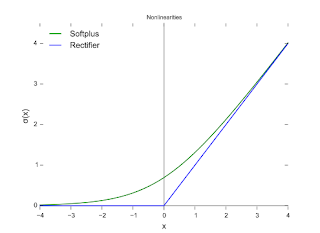We had constructed a NN with just the output layer for classification in the previous post. Now let's insert a layer of ReLUs to make it non-linear. This layer in the middle is called a hidden layer. We now have two matrices. One going from the inputs to the ReLUs, and another one connecting the ReLUs to the classifier.## Backpropagation

If you have two functions where one is applied to the output of the other, then the chain rule tells you that you can compute the derivatives of that function simply by taking the product of the derivatives of the components. $[g(f(x))]' = g'(f(x))*f'(x)$. There is a way to write this chain rule that is very computationally efficient.When you apply your data to some input x, you have data flowing through the stack up to your predictions y. To compute the derivatives, you create another graph that flows backwards through the network, get's combined using the chain rule that we saw before and produces gradients. That graph can be derived completely automatically from the individual operations in your network. Deep learning frameworks will do this backpropagation automatically for you.This backpropagation idea is explained beautifully (I mean it) here.

## Training a Deep NN

So to run stochastic gradient descent (SGD), for every single little batch of data in your training set, the deep NN

• runs the forward prop, and then the back prop and obtains the gradients for each of the weights in the model,
• then applies those gradients to the original weights and updates them,
• and repeats that over and over again until convergence.

You can add more hidden ReLU layers and make your model deeper and more powerful. The above backpropagation and SGD optimization applies the same to deeper NNs. Deep NNs are good at capturing hierarchical structure.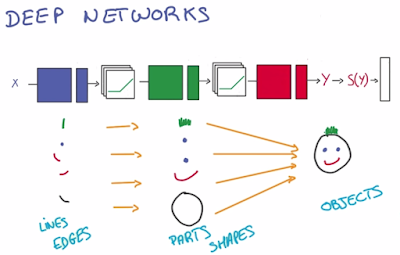## Regularization

In practice, it is better to overestimate the number of layers (and thus model parameters) needed for a problem, and then apply techniques to prevent overfitting. The first way to prevent overfitting is by looking at the performance under validation set, and stopping to train as soon as we stop improving.

Another way is to prevent overfitting is to apply regularization. For example in L2 Regularization, the idea is to add another term to the loss, which penalizes large weights.Another important technique for regularization that emerged recently is the dropout technique. It works very well and is widely used. At any given training round, the dropout technique randomly drops half of the activations that's flowing through the network and just destroy it. (The values that go from one layer to the next are called activations.) This forces the deep NN to learn a redundant representation for everything to make sure that at least some of the information remains, and prevents overfitting.

Here are the links to the introductory ML/DL concepts series:

### Learning Machine Learning: Multinomial Logistic Classification

In the previous post, we got started on classification. Classification is the task of taking an input and giving it a label that says, this is an "A". In the previous post, we covered logistic regression, which made the decision for a single label "A". In this post, we will generalize that to multinomial logistic classification where your job is to figure out which of the K classes a given input belongs to.

For this post I follow the course notes from the Udacity Deep Learning Class by Vincent Vanhoucke at Google. I really liked his presentation of the course: very practical and to the point. This must have required a lot of preparation. Going through the video transcript file, I can see that the material has been prepared meticulously to be clear and concise. I strongly recommend the course.

The course uses TensorFlow to teach you about Deep Neural Networks in a hands-on manner, and follows the MNIST letter recognition example in the first three lessons. Don't get stressed about TensorFlow installation and getting the tutorial environment setup. It is as easy as downloading a Docker container, and going to your browser to start filling in Jupyter Notebooks. I enjoyed programming in the Jupyter Notebooks a lot. Jupyter Notebooks is literate programming ...um, literally.

## Multinomial logistic classification

The logistic classifier takes an input vector X (for example, the pixels in an image), and applies a linear function to them to generate its predictions. The linear function is just a giant matrix multiply: it multiplies X with the weights matrix, W, and add biases, b, to generate its prediction to be one of the output classes.

Of course, we need to first train our model (W and b that is) using the training data and the corresponding training labels to figure out the optimal W and b to fit the training data. Each image, that we have as an input can have one and only one possible label. So, we're going to turn the scores (aka logits) the model outputs into probabilities. While doing so, we want the probability of the correct class to be very close to one and the probability for every other class to be close to zero.

This is how multinomial logistic classification generalizes logistic regression.

• We use a softmax function to turn the scores the model outputs into probabilities.
• We then use cross entropy function as our loss function compare those probabilities to the one-hot encoded labels.

## Softmax function and one-hot encoding

A softmax function, S, is of the form $S(y_i)=\frac{e^{y_i}}{\sum e^{y_j}}$. This way S can take any kind of scores and turn them into proper probabilities which sum to 1.
def softmax(x):
"""Compute softmax values for each sets of scores in x."""
return np.exp(x)/np.sum(np.exp(x), axis=0)

Compare the softmax with the logistic function $g(z)= \frac{1}{(1 + e^{-z})}$ in the logistic regression post. The logistic function was concerned with deciding if the output is label "A" or not (less than 0.5 and it is not A, and more than 0.5 it is A), whereas the softmax function is giving/distributing probabilities for the output being in each of the output class "A", "B", "C", etc., the sum of which adds up to 1.

One-hot encoding is a way to represent the labels mathematically. Each label will be represented by a vector of size output classes and it has the value 1.0 for the correct class and 0 every where else.

## Cross entropy

We can now measure the accuracy of the model by simply comparing two vectors: one is the softmax vector that comes out of the classifiers and contains the probabilities of the classes, and the other one is the one-hot encoded vector that corresponds to the label.

To measure the distance between those two probability vectors, *cross-entropy* is used. Denoting distance with D, Softmax(Y) with S, Label with L, the formula for cross-entropy is: $D(S,L)= -\sum_i L_i log(S_i)$.

When the $i$th entry corresponds to the correct class, $L_i=1$, and the cost (i.e., distance) becomes -log(S_i). If $S_i$ has a larger probability close to 1, the cost becomes lower, and if $S_i$ has a lower probability close to 0, the cost becomes larger. In other words, the cross entropy function penalizes $S_i$ for the false-negatives. When the $i$th entry corresponds to one of the incorrect classes, $L_i=0$ and the entry in $S_i$ becomes irrelevant for the cost. So the cross entropy function does not penalize $S_i$ for the false positives.

Compare the cross-entropy with the cost function in logistic regression:It looks like the cross-entropy does not take into account false-positives, whereas the earlier $J$ cost function took both into account and penalized both the false-positives and false-negatives. On the other hand, cross-entropy does consider false-positives in an indirect fashion: Since the softmax is a zero-sum probability classifier, improving it for the false-negatives does take care of the false-positives.

## Minimizing Cross Entropy via Gradient Descent

To transform the multinomial classification problem into a proper optimization problem, we define training loss to measure the cross-entropy averaged over the entire training sets for all the training inputs and the corresponding training labels: $\mathcal{L} = 1/N * \sum_i D( S(Wx_i+b), L_i)$

We want to minimize this training loss function, and we know that a simple way to do that is via gradient descent. Take the derivative of your loss, with respect to your parameters, and follow that derivative by taking a step downwards and repeat until you get to the bottom.

As we discussed before, in order to speed up gradient descent, normalization is important. Normalization is simple, if you are dealing with images. You can take the pixel values of your image, they are typically between 0 and 255. And simply subtract 128 and divide by 128. W and b should also be initialized for the gradient descent to proceed. Draw the weights randomly from a Gaussian distribution with mean zero and a small standard deviation sigma.

Computing gradient descent using every single element in your training set can involve a lot of computation if your data set is big. And since gradient descent is iterative, this needs to get repeated until convergence. It is possible to improve performance by simply computing the average loss for a very small random fraction of the training data. This technique is called stochastic gradient descent, SGD. SGD is used a lot for deep learning because it scales well with both data and model size.

How small should an SGD step (aka "learning rate") be? This is an involved question: setting the learning rate large doesn't make learning faster, instead using large steps may miss the optima valley, and may even cause divergence. To set a suitable value for learning rate, we can try a range of values 0.001, 0.003, 0.01, 0.03. 0.1, 0.3, and plot convergence. After you settle on a suitable step size to start with, another useful thing is to make the step smaller and smaller as the training progresses during a training run, for example by applying an exponential decay. AdaGrad helps here. AdaGrad is a modification of SGD that makes learning less sensitive to hyperparameters (such as learning rate, momentum, decay).

## How do we go deep?

We devised a neural network (NN) with just 1-layer, the output layer. Our
1-layer NN works like this:

• It multiplies training data by W matrix and adds b
• It applies the softmax and then cross entropy loss to calculate the average of this loss over the entire training data.
• It uses SGD to compute the derivative of this loss with respect to W and b, and applies the $\delta$ adjustment to W and b (i.e., takes a step downwards in the gradient field)
• It keeps repeating the process until it converges to a minimum of the loss function.

In the next post, we will learn about adding hidden layers via rectified linear units (ReLUs) to build deeper NNs.  Deeper NNs are able to capture more complex functions to fit the data better. For training the deep NN we will learn about how to backpropagate the gradient descent adjustments to the corresponding layers in the NN using the chain rule of derivation.

Here are the links to the introductory ML/DL concepts series:

## Friday, January 6, 2017

### Learning Machine Learning: Logistic Regression

This is part 2 of learning machine learning introductory concepts. Recall that supervised learning had two basic examples, regression and classification. We covered linear regression in part 1, and now in part 2 we look at classification. Although the name of the technique used here, logistic regression, includes the word "regression", this is in fact a classification algorithm. It builds on a similar gradient descent approach as we discussed in part 1 in the context of linear regression.

(In this post, again I follow/summarize from Andrew Ng's machine learning course at Coursera. Here is Ng's course material for CS 229 at Stanford. There are also good course notes here, and I will summarize even more briefly than those notes to highlight only the big ideas.)

## Hypothesis representation

The goal of the logistic regression algorithm is to determine what class a new input should fall into. Here is an example application. See, line fitting does not make sense for this application. We need discrete classification into yes or no categories.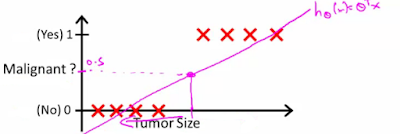For linear regression, our hypothesis representation was of the form $h_\theta(x) = (\theta x)$. For classification, our hypothesis representation is of the form $h_\theta(x) = g((\theta x))$, where we define $g(z)= \frac{1}{(1 + e^{-z})}$. This is known as the sigmoid function, or the logistic function. For a real value $z$, the logistic function has the following plot.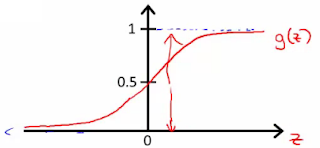If $z$ is positive, $g(z)$ is greater than 0.5. In our logistic regression hypothesis, we take $z = (\theta x)$, so when  $\theta x \geq 0$, then $h_\theta \geq 0.5$ and the hypothesis predicts $y=1$. When $\theta x \leq 0$ then the hypothesis predicts $y=0$.

In other words, $\theta x \geq 0$  is the decision boundary. When our hypothesis $h_\theta(x)$ outputs a number, we treat that value as the estimated probability that y=1 on input x.

If our hypothesis is linear, of the form $h_\theta(x) = g(\theta_0 + \theta_1 x_1 + \theta_2 x_2)$, the decision boundary would be a line. For example: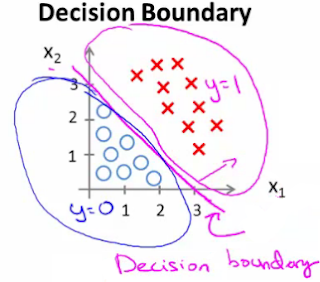If our hypothesis is polynomial, $h_\theta(x) = g(\theta_0 + \theta_1 x_1 + \theta_2 x_1^2 + \theta_3 x_2^2)$ , the decision boundary can be a circle. (By using higher order polynomial terms, you can get even more complex decision boundaries.) For example: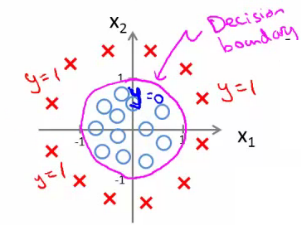OK, assuming we had decided on our hypothesis, how does the logistic regression algorithm learn values for fitting $\theta$ to the data to capture the data nicely in the decision boundary? We again use gradient descent, but this time a little differently as follows.

## Cost function for logistic regression

Since $h_\theta(x) = h_\theta(\frac{1}{(1 + e^{-x})})$ is a sigmoid/nonlinear function, when we plug this in the cost function, we don't know if the cost function will be convex or not.  However, the cost function should be convex for the gradient descent to work. So we use a trick, we define our cost function carefully to make sure when $h_\theta(\frac{1}{(1 + e^{-x})})$  is plugged in the cost function, the function is still a convex function.

We define our cost function as:Note that:
cost (1)= 0 if y=1, else it is infinity
cost (0)=0 if y=0, else it is infinity

In other words, this cost function harshly penalizes and thus aims to rule out very confident mislabels; mislabels can still have lukewarm 0.6 confidence because the penalty is less there.

The above is the cost for a single example. For binary classification problems y is always 0 or 1, and using this, we can have a simpler way to write the cost function, and compress it into one equation as follows.## Gradient descent for logistic regression

We use gradient descent to minimize the logistic regression cost function. As described before the gradient descent algorithm repeatedly does the following update $\theta_j := \theta_j - \alpha \frac{\partial}{\partial \theta_j} J(\theta)$,

where $\frac{\partial}{\partial \theta_j} J(\theta)= \sum_{i=1}^m (h_\theta (x^i)-y^i)*x_j^i$.

## Multiclass classification problems

We can adopt this singleclass logistic regression idea for solving a multiclass classification problem using one vs. all approach: To do k classifications, split the training set into k separate binary classification problems.Here are the links to the introductory ML/DL concepts series:

## Thursday, January 5, 2017

### Learning Machine Learning: Introduction and Linear Regression

In an earlier post, I had talked about how I went about learning about machine learning and deep learning (ML/DL), and said that I would write brief summaries of the introductory ML/DL concepts I learned during that process. I will do part 1 now, otherwise soon I will start to find the introductory concepts obvious and trivial (which they are not). So for all it is worth, and mostly to keep my brain organized, here is the first post on the introductory ML/DL concepts.

## Supervised and Unsupervised Learning Algorithms

Machine learning algorithms are divided broadly into two parts: supervised and unsupervised learning algorithms.

In supervised learning, there is a training phase where a supervisor trains the algorithm with examples of how the output relates to the input. Two basic examples of supervised learning are regression, which uses a continuous extrapolation function for output prediction, and classification, which outputs a classification into buckets/groups. The rest of this post delves into supervised learning via regression. Supervised learning via classification will be the topic of my next learning machine learning post.

(Here is a brief word on unsupervised learning for completeness sake. Unsupervised learning does not have a supervised training phase using labeled training data. Even without any labeled training data to compare the output with, we can still do useful work: we can learn some relations among the input data and classify/cluster the input data into groups. So clustering algorithms are a basic example of unsupervised learning category. I won't be mentioning unsupervised learning for the rest of the post, and probably a good while in the future.)

In the rest of this post, I follow/summarize from Andrew Ng's machine learning course at Coursera. (Here is Ng's course material for CS 229 at Stanford.) There are also good course notes here, and I summarize even more briefly than those notes to highlight the big ideas.

## Linear Regression

Linear regression is a basic supervised learning problem for regression. A canonical application for linear regression is learning house pricing via using existing house pricing data by inferring how the sales price of the houses relates to the number of rooms, square-footage, and the location of the houses.

This is how linear regression works. The algorithm outputs a function: hypothesis, denoted as h. For example, $h= \theta_0 + \theta_1 * x$. The output, y, is given by h(x), which is a linear function of x, the input. The parameters $\theta_0$ and $\theta_1$ are calculated by the linear regression algorithm using gradient descent.

To calculate $\theta_0$ and $\theta_1$, linear regression uses the cost function approach.  To this end, we rewrite the problem as a minimization of error/cost problem. We define cost "$J$" as $(h_θ (x)-y)^2$, and figure out which assignment to θ (i.e., $\theta_0$ and $\theta_1$, also known as the model parameters) gives the minimum error/cost for the training data. $J (θ_0, θ_1) = 1/2m * \sum_{i=1}^m (h_θ (x_i)-y_i)^2$

OK, now that we have the cost function $J(\theta_0, \theta_1)$, how do we go about calculating the $\theta$ parameters that minimize the error/cost for the training data? What technique do we use? We let the error/cost function (also known as "loss") be our guide, and perform a locally (myopically) guided walk in the parameter space towards the direction where the error/cost function is reduced. In other words, we descent on the gradient of the error/cost function.More specifically, we look at the slope of the cost function and descend the gradient with step sizes of $\alpha$. Iterating like this, we eventually(?) hit a local minima, which for a convex cost function/shape is also the global minima.

More concretely, to compute $\theta_0, \theta_1$ that minimizes cost function $J (\theta_0, \theta_1)$, we do the following until convergence: $\theta_j = \theta_j - \alpha \frac{\partial}{\partial \theta_j} J (\theta_0, \theta_1)$.

Here is an example with $\theta_0, \theta_1$. The cost function $J$ is a circle/oval. (If there were only $\theta_1$, $J$ would be a line. If there were $\theta_k$, for $k>3$, $J$ would be hard to draw.)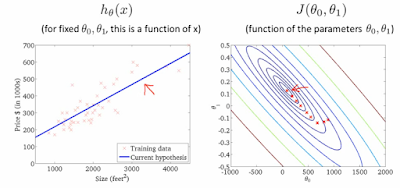Here $\alpha$ is the learning rate. While calculating $\theta_j$, we update simultaneously for $\theta_0$ and $\theta_1$.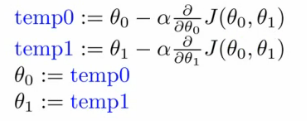Too small an $\alpha$ would mean that convergence takes a long time. Too big an $\alpha$ may lead to missing convergence and even divergence. To set a suitable value for $\alpha$, we can explore and identify an $\alpha$ that is good enough. To do this we can try a range of alpha values 0.001, 0.003, 0.01, 0.03. 0.1, 0.3, and plot $J(\theta)$ vs number of iterations for each version of $\alpha$. What can I say, ML is a very empirical field of study.

## Linear regression with multiple features

Lets talk about how to generalize linear regression from the linear regression with 1 feature we considered above. Here we make $\theta$ and $x$ into a vector and the algorithm is the same as that of linear regression. Here is the generalized algorithm:If you have a problem with multiple features, you should make sure those features have a similar scale. If not, the circle (or more accurately the multidimensional spherical shape) could be dominated by one feature $\theta_j$, and would have a very slanted/elongated oval shape rather than a nice circle. And that will prevent the gradient descent to converge quickly to the eye of the target as it will spend too much time walking through the elongated oval.

For feature scaling we can employ mean normalization: Take a feature $x_i$, Replace it by ($x_i$ - mean)/max. Now your values all have an average of about 0.

## Conclusion

This was mostly basic line fitting by relying on trial and error. But this a primitive on which the rest of the Ml/DL (machine learning / deep learning) work builds on. In the next post on learning machine learning series, we will look at supervised classification problems and then use that to start learning about neural networks and deep learning.